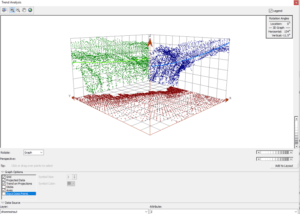### Exploratory data analysis for geostatics: trend analysis

Following the article   Introduction to  Exploratory data analysis for geostatistics   we will discuss each of the available tools to perform the exploratory analysis of the spatial data. We have discussed the  histograms ,    QQ-Plots , and Voronoï maps . Now, it is the turn for the data trends.

Trend? What is it?

Firstly we have to know what we are looking for. Certainly, you are already familiar with the notion of trend in the temporal series (the trend of unemployment is decreasing or increasing, etc …).

The topic here is spatial data processing. Therefore the notion of trend is a little different since we do not have, a priori, the variable time to organize our points in a temporal series.

Let’s use the example used in the previous articles, a series of points resulting from the use of a bathymetric sensor.Remember what we said in a previous article Introduction on the basics of geostatistical: it deals with random phenomena random with dependency. Are we entitled to assume that in the considered area the depths are distributed in our study area so randomly? Of course not. We know that depth will increase, in principle, as we move away from the coast. If we draw a line off the coast :and that we project the value of our points on the plane defined by this line :we notice that indeed , the cloud of our points shows a relationship between the distance to the coast and the depth observed .

That is what we will call a trend in our spatial data: when a function is verified between the values of the points and a given direction in the three spatial axes.

What to do and why.

If you apply a geostatistical method (kriging, for example) on the data that shows a trend , you are not respecting the basic assumptions of geostatistics . The phenomenon that is going to stand out concealing any other phenomenon is the distance to the flank. You are going to mess around with a complicated tool to get a result that will not be better than the one you could get with a deterministic method  (IDW, Spline , …) much simpler to execute .

If you are interested in that trend, forget the geostatics and use the classic deterministic interpolating methods.

However, if you are interested in researching whether there are more subtle phenomena that determine the depths:The midpoint between the two black bars is explained by the distance to the coast. But, the dispersion of values for each distance, can be modelled or not? Here is where geostatistics comes into play:  if you want to, not only, forecast the values as a function of distance to the coast, but also, as function of those phenomena invisible at first sight.

Trend analysis

The Geostatistical Analyst tool to analyse the trend can be helpful to identify global trends in the entry data set.
This tool   provides a three – dimensional view of the data. The various locations of the sampling points are plotted on the x, y plan. Besides,  for each sampling point, the value is defined by the height   in the z dimension   . The interest of the trend analysis tool lies in the fact that the values are next projected on the x, z and y plans, z depicts the scatter plots.This can be considered as lateral views through the three-dimensional data. Then the polynomials are adjusted through the scatterplots on the projected plans.

By applying rotations on the different axes you can observe the result live, on the points cloud projected onto each plane as well as the adjustment polynomial calculated curve.This returns to off the coast straight line of our example and turn it in all the directions in order to figure out which is the most marked trend.

By rotating the axes, we get to see both adjustment curves. The blue curve adjusts very closely the cloud of blue dots, for a given orientation. In our example, the green curve, perpendicular to the blue curve, does not indicate any visible trend.

Trendautocorrelation and nugget effect

A surface can be composed of two main components: a   fixed global trend    and a   short range random variation.

The global trend is frequently called fixed average structure. Short range random variation (also called random error) can be modelled in two parts: spatial autocorrelation and nugget effect. These two notions are used when modelling the semi-variogram.

If you identify a global trend in your data, then you must decide how to model it. Whether you will use a deterministic or geostatic method to create a surface depends of your final objective. If you only want to model the global trend and create a smooth surface, you can use a local or global interpolating polynomial method to perform a final interpolation.

However, you can handle the trend with a geostatistical method. First of all, we have to delete the trend, by subtracting the trend at each point and by keeping the residue as inputs of the geostatistical method.

Then, we model the residues (the remaining component) as short range random variations by using a Kriging method.

When we obtain the result, we must add the result to each mesh, the value of the calculated trend for this mesh .

The main reason to delete a trend in geostatistics is to satisfy the stationary hypothesis.

If you break down your data in trend plus the short reach variation, you suppose the trend is fixed and that short range variation is random. Here, random does not mean ”unpredictable” but rather what is ruled by the probability laws that include dependence on neighbouring values otherwise called autocorrelation .

The final surface will be the sum of the fixed and random surfaces. In other terms, think that you will add two layers to achieve the final result: that which never changes (trend), and one that changes randomly (autocorrelation).

If you can identify and quantify the trend, you will get a deeper understanding of your data and, therefore, you will be able to make better decisions. If you remove the trend, you will be able to model more accurately the random variation at short range because the global trend  will not influence your spatial analysis .

Examination of the trend using global trend analysis

The geostatistical analyst Trend Tool projects the points in two directions (default, north and east) on plans perpendicular to the map plan.

A polynomial curve is adapted to each projection. The entire surface of the map can be turned in anything independent of the direction, which also amends the direction represented by the projected plans. If the curve through the projected points is flat, no trend exists, as shown in the green line in the plan projected to the left in the above image.

If the polynomial is defined as a  curved model (up or down, as shown with the  blue line in the projected left plan in the above diagram ), this suggests a trend  in the global data.

It suggests that a second- order polynomial can be adapted to the data. Thanks to the refinement granted by the trend analysis tool, we can identify the real orientation of the trend. In this case, the strongest influence is from north-east to south-west.

Trend order

Once you have detected a trend, you still have to define its order (first, second , third , …). Even if it seems complicated, it is very simple.

Take a sheet of paper.

Keep it right and incline it.  If your trend looks like that, you have a first order trend. At the tool trend geostatistical analyst level it translates as adjustments in two straight lines .

Bend the sheet so as to create a hollow and a hump. If your tendency to this shape, you have a second order trend. At the geostatistical analyst tool trend this translates into an adjustment as two curves, each one with its own curvature.

And so on. You simply must count the number of bends you find and add 1, to have the order of the polynomial.

## 2 thoughts on “Exploratory data analysis for geostatics: trend analysis”

1.aradhana says:

sir,
is it possible to use 30 years .nc format data (1 file/ years) for trend detection? if yes than how ?

1.Atilio Francois says:

Sorry, but you’re confusing two different concepts:
-trend analysis in time series, and
-Trend analysis in geographic data;
The article deals with the trend of the points values that you want to make a continuous map of.